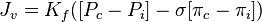## Starlings equation and starlings forces.

Starling equation is:• ([PcPi] − σ[πc − πi]) is the net driving force,
• Kf is the proportionality constant, and
• Jv is the net fluid movement between compartments.

By convention, outward force is defined as positive, and inward force is defined as negative. The solution to the equation is known as the net filtration or net fluid movement (Jv). If positive, fluid will tend to leave the capillary (filtration). If negative, fluid will tend to enter the capillary (absorption).

Starlings forces are the major determinants in the development of edema.
According to Starling’s equation, the movement of fluid depends on six variables:

1. Capillary hydrostatic pressure ( Pc )
2. Interstitial hydrostatic pressure ( Pi )
3. Capillary oncotic pressure ( πc )
4. Interstitial oncotic pressure ( πi )
5. Filtration coefficient ( Kf )
6. Reflection coefficient ( σ )

Capillary hydrostatic pressure-Pushes fluid out from the capillaries
Capillary Oncotic pressure-Pulls fluid in
Interstitial hydrostatic pressure-pushes fluid into the venous end
Interstitial oncotic pressure-pulls fluid out